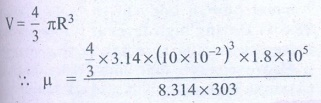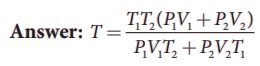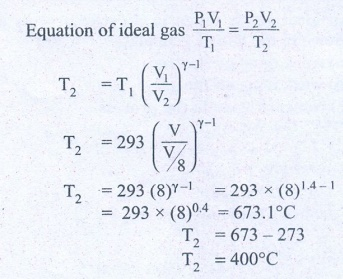Home | Heat and Thermodynamics: Important Questions

# Heat and Thermodynamics: Important Questions

1. ‘An object contains more heat’- is it a right statement? If not why?

2. Obtain an ideal gas law from Boyle’s and Charles’ law.

3. Define one mole.

4. Define specific heat capacity and give its unit.

5. Define molar specific heat capacity.

6. What is a thermal expansion?

7. Give the expressions for linear, area and volume thermal expansions.

8. Define latent heat capacity. Give its unit.

9. State Stefan-Boltzmann law.

10. What is Wien’s law?

11. Define thermal conductivity. Give its unit.

12. What is a black body?

13. What is a thermodynamic system? Give examples.

14. What are the different types of thermodynamic systems?

15. What is meant by ‘thermal equilibrium’?

16. What is mean by state variable? Give example.

17. What are intensive and extensive variables? Give examples.

18. What is an equation of state? Give an example.

19. State Zeroth law of thermodynamics.

20. Define the internal energy of the system.

21. Are internal energy and heat energy the same? Explain.

22. Define one calorie.

23. Did joule converted mechanical energy to heat energy? Explain.

24. State the first law of thermodynamics.

25. Can we measure the temperature of the object by touching it?

26. Give the sign convention for Q and W.

27. Define the quasi-static process.

28. Give the expression for work done by the gas.

29. What is PV diagram?

30. Explain why the specific heat capacity at constant pressure is greater than the specific heat capacity at constant volume.

31. Give the equation of state for an isothermal process.

32. Give an expression for work done in an isothermal process.

33. Express the change in internal energy in terms of molar specific heat capacity.

34. Apply first law for (a) an isothermal (b) adiabatic (c) isobaric processes.

35. Give the equation of state for an adiabatic process.

36. Give an equation state for an isochoric process.

37. If the piston of a container is pushed fast inward. Will the ideal gas equation be valid in the intermediate stage? If not, why?

38. Draw the PV diagram for

a. Isothermal process

c. isobaric process

d. Isochoric process

39. What is a cyclic process?

40. What is meant by a reversible and irreversible processes?

41. State Clausius form of the second law of thermodynamics

42. State Kelvin-Planck statement of second law of thermodynamics.

43. Define heat engine.

44. What are processes involves in a Carnot engine?

45. Can the given heat energy be completely converted to work in a cyclic process? If not, when can the heat can completely converted to work?

46. State the second law of thermodynamics in terms of entropy.

47. Why does heat flow from a hot object to a cold object?

48. Define the coefficient of performance.

1. Explain the meaning of heat and work with suitable examples.

2. Discuss the ideal gas laws.

3. Explain in detail the thermal expansion.

4. Describe the anomalous expansion of water. How is it helpful in our lives?

5. Explain Calorimetry and derive an expression for final temperature when two thermodynamic systems are mixed.

6. Discuss various modes of heat transfer.

7. Explain in detail Newton’s law of cooling.

8. Explain Wien’s law and why our eyes are sensitive only to visible rays?

9. Discuss the

a. thermal equilibrium

b. mechanical equilibrium

c. Chemical equilibrium

d. thermodynamic equilibrium.

10. Explain Joule’s Experiment of the mechanical equivalent of heat.

11. Derive the expression for the work done in a volume change in a thermodynamic system.

12. Derive Mayer’s relation for an ideal gas.

13. Explain in detail the isothermal process.

14. Derive the work done in an isothermal process

15. Explain in detail an adiabatic process.

16. Derive the work done in an adiabatic process

17. Explain the isobaric process and derive the work done in this process

18. Explain in detail the isochoric process.

19. What are the limitations of the first law of thermodynamics?

20. Explain the heat engine and obtain its efficiency.

21. Explain in detail Carnot heat engine.

22. Derive the expression for Carnot engine efficiency.

23. Explain the second law of thermodynamics in terms of entropy.

24. Explain in detail the working of a refrigerator.

## 1. Calculate the number of moles of air is in the inflated balloon at room temperature as shown in the figure.The radius of the balloon is 10 cm, and pressure inside the balloon is 180 kPa.AnswerThe radius of the balloon R = 10 × 10-2 mThe pressure inside the balloon P = 1.8 × 105 PRoom temperatureT = 303 k (273 + 30)Number of moles of air μ = ?V - volume of air in balloonV=- 4/3 IR3μ = 0.3 molesAnswer: μ ≅ 0.3 mol2. In the planet Mars, the average temperature is around -53°C and atmospheric pressure is 0.9 kPa. Calculate the number of moles of the molecules in unit volume in the planet Mars? Is this greater than that in earth?SolutionAverage temperature of the mass T = -53°CAtmospheric pressure P = 0.9 × 103 PVolume V = 1 m3Number of moles n = ?μmass = VP / RT = 0.9×103 / 8.314x220 = 0.38 molMe = 0.38 mol.Answer: μMars = 0.49 molμEarth ≅ 40 mol3. An insulated container of gas has two chambers separated by an insulating partition. One of the chambers has volume V1 and contains ideal gas at pressure P1 and temperature T1. The other chamber has volume V2 and contains ideal gas at pressure P2 and temperature T2. If the partition is removed without doing any work on the gases, calculate the final equilibrium temperature of the container.Solution:The volume pressure and temperature of the gas in first chamber is V1, P1 & T1By conservation of energy4. The temperature of a uniform rod of length L having a coefficient of linear expansion αL is changed by ∆T. Calculate the new moment of inertia of the uniform rod about axis passing through its center and perpendicular to an axis of the rod.Solution:M.I of uniform rod of mass M. length l about its center & perpendicular isWhen rod is heated by temperature ΔT, there is increase in length of rod ΔlΔl = lαLΔT  ……… (1)(αL - coefficient of linear expression)We get substituting Δl from (1)I' = Ml2 /12 (1+ αL ΔT)2I' = (1+ αL ΔT)2 (from equation (A)Answer: I' = I (1 + αL ∆T)25. Draw the TP diagram (P-x axis, T-y axis), VT(T-x axis, V-y axis) diagram fora. Isochoric processb. Isothermal processc. isobaric process6. A man starts bicycling in the morning at a temperature around 25°C, he checked the pressure of tire which is equal to be 500 kPa. Afternoon he found that the absolute pressure in the tyre is increased to 520 kPa. By assuming the expansion of tyre is negligible, what is the temperature of tyre at afternoon?Answer: T= 36.9°C7. Normal human body of the temperature is 98.6°F. During high fever if the temperature increases to 104°F, what is the change in peak wavelength that emitted by our body? (Assume human body is a black body)Answer: (a) λmax ≈ 9348 nm at 98.6°F (b) λmax ≈ 9258 nm at 104°F8. In an adiabatic expansion of the air, the volume is increased by 4%, what is percentage change in pressure? (For air γ = 1.4)Answer: 5.6%9. In a petrol engine, (internal combustion engine) air at atmospheric pressure and temperature of 20°C is compressed in the cylinder by the piston to 1/8 of its original volume. Calculate the temperature of the compressed air.(For air γ = 1.4)At temperature T,Atmospheric pressure of air = 1 atmInitial volume of air V1= V m3Initial temperature of air T1 = 20°C= 20+273 = 293KAt temperature T2Volume of the air is compressed by a factor of – 1/8The new volume V2 = V. (1/8) m3γ= 1.4The atmospheric pressure of the gas at final temperature T2 = ?Answer: T ≅ 400°C10. Consider the following cyclic process consist of isotherm, isochoric and isobar which is given in the figure.Draw the same cyclic process qualitatively in the V-T diagram where T is taken along x direction and V is taken along y-direction. Analyze the nature of heat exchange in each process.SolutionIn element 1-2, the gas undergoes isothermal expansions. It receiver’s certain amount of heat from the outside of spends all this heat in doing work the internal energy of the gas remains unchanged.In element 2-3, the gas is heated isochorically (at constant volume). Since its volume does not change, no work is done. The internal energy of the gas is increased only due to the heat transferred to the gas from the outside.In element 3-1, the gas is compressed isobarically (at constant pressure) of its temperature drops work is done on the gas, but its internal energy is reduced. This means the gas intensively gives up heat to the medium. Answer: T= 36.9°CProcess 1 to 2 = increase in volume. So heat must be added. Process 2 to 3 = Volume remains constant. Increase in temperature. The given heat is used to increase the internal energy.Process 3 to 1 : Pressure remains constant. Volume and Temperature are reduced. Heat flows out of the system. It is an isobaric compression where the work is done on the system.11. An ideal gas is taken in a cyclic process as shown in the figure. Calculate(a) work done by the gas.(b) work done on the gas(c) Net work done in the processAnswer: (a) W = +1.5kJ(b) W = −1.2kJ(c) W = +300J.12. For a given ideal gas 6 × 105J heat energy is supplied and the volume of gas is increased from 4 m3 to 6 m3 at atmospheric pressure. Calculate (a) the work done by the gas (b) change in internal energy of the gas (c) graph this process in PV and TV diagram.SolutionHeat energy supplied to the gas Q = 6 × 105JChange in Volume ΔV = (6 - 4) = 2m3(a) Work done by the gas W =?Atmospheric pressureP = 1.013 × 105 Nm-2W = P.ΔVW = 1.013 × 105 × 2 = 2.026 × 105 (or) 202.6 kJ(b) Change in internal energy ΔU =?Δu = W = Q - P. ΔV= 6 × 105-2.026 × 105= 3.974 × 105J (or) 397.4kJΔu = 397 kJ.(c)Answer: (a) W = +202.6 kJ(b) dU = 397.4 kJ13. Suppose a person wants to increase the efficiency of the reversible heat engine that is operating between 100°C and 300°C. He had two ways to increase the efficiency. (a) By decreasing the cold reservoir temperature from 100°C to 50°C and keeping the hot reservoir temperature constant (b) by increasing the temperature of the hot reservoir from 300°C to 350°C by keeping the cold reservoir temperature constant. Which is the suitable method?SolutionTemperature of sin x T2 = 100°C + 273 = 373KTemperature of sin x T1 = 300°C + 273 = 573 KEfficiency η = ?Answer: Initial efficiency = 44.5%Efficiency in method (a) =52 %Efficiency in method (b) =48 %Method (a) is more efficient.14. A Carnot engine whose efficiency is 45% takes heat from a source maintained at a temperature of 327°C. To have an engine of efficiency 60% what must be the intake temperature for the same exhaust (sink) temperature?SolutionEfficiency of Carnot engine η1 = 45%Efficiency of Carnot temperature of source T1 = 327°CEfficiency of Carnot temperature of sink T2 = ?= 327 + 273 = 600 KEfficiency of carnot temperature η2 = 60%when efficiency of Carnot temperature of source T =?Answer: 552°C15. An ideal refrigerator keeps its content at 0°C while the room temperature is 27°C. Calculate its coefficient of performance. SolutionContent placed at temperature TL = 0°C + 273 = 273KRoom temperature TH = 27°C + 273 = 300 KCoefficient of performance β = ?β = 10.11Answer: β=10.11

Tags : Physics , 11th Physics : UNIT 8: Heat and Thermodynamics
Study Material, Lecturing Notes, Assignment, Reference, Wiki description explanation, brief detail
11th Physics : UNIT 8: Heat and Thermodynamics : Heat and Thermodynamics: Important Questions | Physics